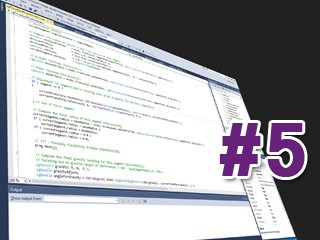# Mathematics for Game Development### Set Theory

Goals: Understand the basics of set theory and its symbols. Learn how entities are grouped into sets. Conduct various operations on sets, such as unions and intersections (i.e., the algebra of sets). Introduce the relationship between functions and sets to set the stage for the next conversation.

• Video Runtime: 20m 4s### Functions

Goals: Understand the role of functions in mathematics and in games. Look at the concept of mapping values between domain and range. Spend time visualizing various kinds of functions using graphs. Discuss some of the popular functions (absolute value, exponential, logarithmic) used in game development. Model game phenomena like fog and weapon damage using functions. Design a custom function and develop a means for selecting appropriate values that reflect the desired outcome.

• Video Runtime: 13m 48s### Polynomials

Goals: Examine the algebra of polynomials. Study graphs for various kinds of polynomial functions. Learn how to apply different kinds of polynomials in game development projects. See how to use linear interpolation to draw polygons on the display. Learn how to use polynomials to approximate the behavior of complex functions too costly to compute in real-time games. Learn how to use polynomials to predict the future values of variables.

• Video Runtime: 16m 44s### Basic Trigonometry I

Goals: Develop a firm grasp of the properties of triangles, and right triangles in particular. Look at the relationships that exist between the internal angles and the lengths of triangle sides. Understand the most commonly used trigonometric functions (sine, cosine, tangent) that relate triangle properties to unit circles. See how to use triangle properties and functions to solve a number of issues related to graphics programming. Create an animated wave function that might be used for a water or cloth simulation. Examine how to use trigonometric concepts to render circles and ellipses on the display.

• Video Runtime: 8m 9s### Basic Trigonometry II

Goals: Look at how inverse trig functions (arcsin, arcos, arctan) can be used to determine angle values. Get comfortable with the core trig identities, such as the reduction and double angle identities. Learn how to use trig identities as a means for deriving mathematical proofs. Use trigonometry to rotate points in two and three dimensions. Construct a proper field-of-view for an in-game camera system.

• Video Runtime: 7m 6s### Analytic Geometry I

Goals: Learn the basics of analytic geometry. Use functions and polynomials to mathematically represent points, lines, planes and ellipses. Understand 2D lines and their various forms, including the all-important parametric representation. Look at intersection and distance formulas with respect to lines, points, and planes. Briefly examine ellipsoidal intersections.

• Video Runtime: 12m 48s### Vectors

Goals: Get very comfortable with vectors, the mathematical heart of all 3D game engines. Learn vector addition and subtraction, scalar multiplication, and the all-important dot and cross products. Examine the use of vectors in games. Discuss the relationship between vectors and planes and the plane representation. Revisit distance calculations using vectors. Learn how to rotate and scale geometry using vector representations of mesh vertices.

• Video Runtime: 7m 58s### Matrices I

Goals: Get very comfortable with matrices, one of the other core components of every 3D game engine. Understand matrices from a purely mathematical perspective. Know what matrices are and what problems they are intended to solve. Examine important matrix mathematics -- addition and subtraction, multiplication by scalars and/or by other matrices. Learn how to use matrices to solve systems of linear equations with Gaussian elimination.

• Video Runtime: 6m 24s### Matrices II

Goals: Understand the idea of linear transformations. Learn how 4D homogenous coordinates can make the non-linear translation operation compliant with matrix operations. Examine a number of common matrices used to effect transformations in 3D games (projection, rotation, translation, scaling, skewing). See how to perform rotations around all three coordinate axes. Study the vector/matrix transformation operation (multiplication) at the heart of all 3D graphics rendering pipelines.

• Video Runtime: 11m 10s### Quaternions

Goals: Examine the concept of imaginary numbers and the various arithmetical operations that can be performed on them. Discuss the similarities and differences between imaginary and real numbers. Study complex numbers and the algebra involved in working with them. Examine the quaternion and its associated algebra. Look at applications of the quaternion in game development. Understand how to use quaternions to perform rotations about arbitrary axes. Solve the gimbal lock problem encountered with Euler angles. Create an updated world-to-view space transformation matrix that is derived from a quaternion after rotation has taken place.

• Video Runtime: 9m 31s### Analytic Geometry II

Goals: Spend time focusing on some practical applications of mathematics in games. Look at how analytic geometry plays an important role in a number of different areas of game development. Learn how to design a simple collision/response system in 2D using lines and planes (e.g., a billiards simulation). Find a way to detect collision between two convex polygons of arbitrary shape. Use vectors and planes to create reflections such as might be seen in a mirror. See how to use of a convex volume to create shadows in a game world. Take a look how vector dot products can be used to determine the lighting and shading of points across a surface.

• Video Runtime: 8m 46s